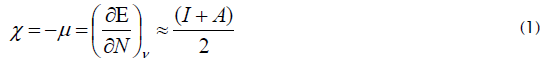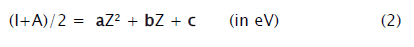e-ISSN: 2319-9849

All submissions of the EM system will be redirected to Online Manuscript Submission System. Authors are requested to submit articles directly to Online Manuscript Submission System of respective journal.

# Electronegativity: An Insight in Terms of Atomic Number Approached Via Isoelectronic Series

Dinesh Prakash Upadhyay*, and Mohan Kumar Sharma

Department of Chemistry, MSJ College, Bharatpur, Rajasthan 321001, India

*Corresponding Author:
Department of Chemistry
MSJ College, Bharatpur
Rajasthan 321001, India

Received: 02/03/2013 Revised: 17/03/2013; Accepted: 20/04/2013

Visit for more related articles at Research & Reviews: Journal of Chemistry

## Abstract

In this communication, an insight into electronegativity in terms of fundamental property, the atomic number (Z) is approached via isoelectronic series, using the electronegativity measure, (I+A)/2 (isoelectronic values) vs. atomic number plots. On the basis of these excellent smooth quadratic curves (r2≈1), we have inferred that the neutral atom electronegativity, (I+A)/2 is proportional to Z2. Our view justifies the suggestion made by Islam et al.

#### Keywords

Insight, Atomic number, Isoelectronic series, Electronegativity.

#### Introduction

Electronegativity [1,2] has become an indispensible tool for chemist, to understand physico-cs properties. This theoretical construct of chemistry finds multifarious applications in biology, physics and geology [3,4], nanochemistry, hightemperature superconductors [5,6], spectroscopy [7,8], qualitative understanding of quark atoms  and several others.

Pauling [1,2] defined electronegativity, “as the power of an atom in a molecule to attract electrons to itself.” Pauling , Mulliken , Gordy , Allred and Rochow  are some of the pioneering measures of electronegativity. Besides these, there are other scales as well [3,4]. In density functional theory (DFT) [13,14], the electronegativity (χ) is defined [15,16] as followsWhere, ν is external nuclear potential and E, is the total energy of an N-electron system. The approximate expression for electronegativity in terms of ionization potential, I, and electron affinity, A provides a reliable electronegativity measure. It is also the Mulliken electronegativity measure .

The privilege of correlations that electronegativity enjoys with large number of atomic and molecular properties such as atomic radius , ionization potential , polarizability  etc. click and reflect something. In atomic species, most of the properties are governed by the electron distribution around the nucleus. So, they have common origin: the atomic nucleus or the atomic number, and the surrounding electrons. It is the binding of the surrounding electrons by the nucleus that is an important controlling factor for most of the properties. Therefore, an insight of electronegativity in terms of atomic number is required and in this communication, this has been done via isoelectronic series.

Isoelectronic series are fundamental periodic properties . Isoelectronic species possess equal number of electrons. Therefore, the change in any property will be mainly due to atomic number. Recently, we have reported the variation of Mulliken’s electronegativity measure, (I+A)/2 isoelectronic values with atomic number . However, nothing specifically approaching the electronegativity of neutral atomic species has been pointed out. In the present work, we identify interrelation between electronegativity of neutral atoms and their atomic number, using isoelectronic series as a tool. This has been achieved through an analysis of the variation of isoelectronic values of (I+A)/2 with atomic number, Z. In the present study, we have analysed the first ten isoelectronic series (H-Ne).

#### Methodology

The methodology that we here use is very simple. It is based on the fact that every neutral atomic species is also a part of some isoelectronic series (series, hereafter). Therefore, from the (I+A)/2 (isoelectronic values) vs. atomic number plots, we can infer about the interrelation between the two i.e electronegativity and atomic number, as for neutral atomic species, (I+A)/2 is the electronegativity. A strict adherence of I and A as the first ionization potential and electron affinity is not required. We just need consecutive ionization potentials that can be depicted as I and A.

#### Results

The electronegativity measure, (I+A)/2 (isoelectronic values) vs. atomic number (up to Z= 20) plots, for the first ten isoelectronic series, are presented in Figures 1-2. These plots  can be represented by (2)

Figure 1: The electronegativity measure, (I+A)/2 vs. atomic number plots (reproduced with permission), for H (1s1), and He (1s2) like isoelectronic series. The solid lines are least square line. The (I+A)/2 values computed using successive ionization potentials from reference .

Figure 2: The electronegativity measure, (I+A)/2 vs. atomic number plots for Li (1s22s1), Be (1s22s2), B (1s22s22p1), C (1s22s22p2), N (1s22s22p3), O (1s22s22p4), F (1s22s22p5) and Ne (1s22s22p6) like isoelectronic series. The solid lines are least square line. The (I+A)/2 values computed using successive ionization potentials from reference .In Table 1 the determination coefficients, r2 and correlation coefficients a, b, and c, of the Figures 1-2 plots, obtained from least square fitting, are listed.

Table 1: The determination coefficients, r2, and correlation coefficients a, b, c, of the variations of electronegativity measure, (I+A)/2 with atomic number (Figure 1-2, Eq. 2), for the first ten isoelectronic series (H-Ne).

All the ionization potentials that we have used, for (I+A)/2 computation, are from reference .

#### Discussion

For the first time an insight into electronegativity in terms of atomic number is approached via isoelectronic series. It can be seen from Figure 1-2 that the plots are apparently smooth curves and strikingly, the data are not scattered. Equation (2) points towards a quadratic relationship. It reveals from the determination coefficients (r2=1), listed in Table 1, that there is high degree of correlation between the electronegativity measure, (I+A)/2 (isoelectronic values) and atomic number, Z. The exact depiction of variation of Figure 1-2 is Eq. (2) expressed through Table 1. On this basis, we infer (as the neutral atomic species are also part of some isoelectronic series) and suggest that the electronegativity is proportional to Z2, provided (I+A)/2 is the measure.

Our present inference and methodology justify the suggested proportionality between electronegativity and square of the effective nuclear charge (Zeff) .

#### Conclusion

An insight of electronegativity in terms of atomic number, approached via isoelectronic series, reveals that if (I+A)/2 is the measure, the electronegativity is proportional to square of the atomic number. The present work justifies the suggested proportionality between electronegativity and square of the effective nuclear charge.

#### Acknowledgement

One of the authors (DPU) is grateful to university grants commission (UGC) for a fellowship.

Replica watches